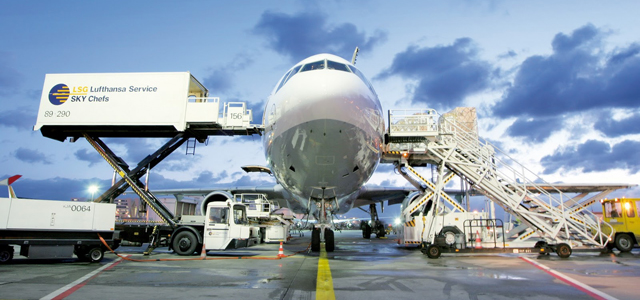# Air Freight Charge Calculation System for Garments Import-Export

By | July 5, 2015

### Chargeable Weight Calculation System Under Air Freight for Garments Import-Export

Mayedul Islam
Merchandiser at Fashion Xpress Buying House.
Email: [email protected]

#### Introduction:

Normally freight charge under air shipment of garments is totally depends on both gross weight or actual weight and cargo weight. If the gross weight or actual weight is higher than cargo weight, then freight charge is fixed on the basis of gross weight. Again, if cargo weight is higher than gross weight, then freight charge is fixed on the basis of cargo weight. That means, among two weights, gross weight and cargo weight, which one will be higher, freight charge will be fixed on that.Garments Import-Export with Air

Chargeable weight is an equilibrium point, where actual weight and cargo weight are totally balanced. But there is an important reason that why freight charge under air shipment is totally depends on both gross weight or actual weight and cargo weight.

One example is enough to remove the confusion about it.
Suppose, you export 500kg cotton and another one export 500kg iron under air shipment. Your exported cotton needed more space than iron, but weight is the same. In this case, if air freight is charged on the basis of cargo weight (on the basis of space) then you has to pay more amount of charge than iron exported person. As a result, actual weight or gross weight and cargo weight both are in consideration during freight charge, which one is higher, that’s chosen for freight charge.

So, chargeable weight calculation system in air freight is discussed in the following:
At first you have to find the cargo measurements with length, width and height. Then calculate the CBM of cargo by using following formula-

CBM calculation for cargo = Length (m) ×weight (m) × height (m)

After calculating CBM of cargo, it’s divided by 0.006. This result is termed as cargo weight. Now, cargo weight is compared to the gross weight or actual weight. Which one is higher, will consider as a chargeable weight.

Now I will provide two examples to remove the all confusion about the above explanation.

#### Example-01:

Suppose,
Gross weight or actual weight is 200kg,

• Cargo length is 100cm = 1m,
• Cargo width is 100cm = 1m,
• Cargo height is 100cm = 1m.

Find out the chargeable weight for air freight of this shipment.

Solution:
Now,
We calculate the Cargo CBM = Length (m) ×weight (m) × height (m)
= (1 × 1 × 1) m3
= 1m3

Now, cargo CBM has to divide by 0.006,

Cargo weight = Cargo CBM (m3)/ 0.006
= 1/ 0.006
= 166.67kg.

So, the gross weight or actual weight is 200kg and cargo weight is 166.67kg. Among two weights, gross weight or actual weight is higher than cargo weight. As a result gross weight or actual weight will be chosen for chargeable weight for this shipment.

#### Example-02:

Suppose,
Gross weight or actual weight is 140kg,

• Cargo length is 98cm = 0.98m,
• Cargo width is 95cm = 0.95m,
• Cargo height is 96cm = 0.96m.

Find out the chargeable weight for air freight of this shipment.

Solution:
Now,
We calculate the Cargo CBM = Length (m) ×weight (m) × height (m)
= (0.98 × 0.95 × 0.96) m3
= 0.89376 m3

Now, cargo CBM has to divide by 0.006,

Cargo weight = Cargo CBM (m3)/ 0.006
= 0.89376/ 0.006
= 148.96kg.

In this shipment, the gross weight or actual weight is 140kg and cargo weight is 148.96kg. Among two weights, cargo weight is higher than gross weight or actual weight. As a result cargo weight will be chosen for chargeable weight for this shipment.

## 3 thoughts on “Air Freight Charge Calculation System for Garments Import-Export”

1.lingaraj.b.moji

Very informative
can we know how to calculata the costing of the fabric for a buyer for negitiating the price

1.Azgor hossain

Very very thanks…………..sir.
Most important…………valuable.

2.GB Linkhon

Dear Maydul vi,
Please give me yarn document tarms 7 detail

This site uses Akismet to reduce spam. Learn how your comment data is processed.